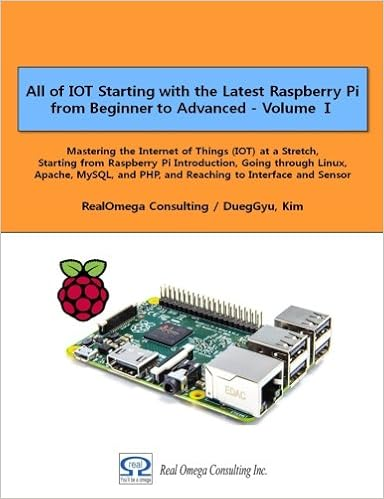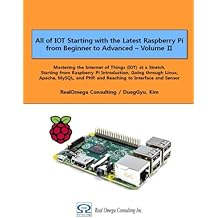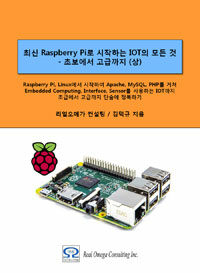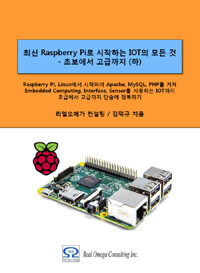# Raspberry Pi_Eng_25.4.6 Direct Current Power

#### Published Book on AmazonAll of IOT Starting with the Latest Raspberry Pi from Beginner to Advanced – Volume 1All of IOT Starting with the Latest Raspberry Pi from Beginner to Advanced – Volume 2

#### 출판된 한글판 도서최신 라즈베리파이(Raspberry Pi)로 시작하는 사물인터넷(IOT)의 모든 것 – 초보에서 고급까지 (상)최신 라즈베리파이(Raspberry Pi)로 시작하는 사물인터넷(IOT)의 모든 것 – 초보에서 고급까지 (하)

### 25.4.6Direct Current Power

The power of electricity is called electric power. The electric power is equal to the value of multiplying a voltage by a current. Electric power means the amount of energy consumed when a current goes through a resistance. The electric power is expressed in W (Watt).

The electric power at direct current is calculated as follows.

Electric Power = Voltage × Current     ( P = VI )

Then, since V = I R in the Ohm's law, substituting this into the equation above, the electric power can be expressed as follows. Here, we can calculate the electric power with the current and the resistance.

P = VI = (I R) x I = I2 x R  [W]

And since I = V / R, the electric power can also be expressed as follows. Here, we can to calculate the electric power with the voltage and the resistance.

P = VI = V x (V / R) = V2 / R  [W]